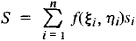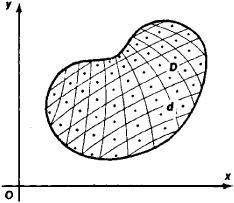# Multiple Integral

(redirected from Area integral)

## multiple integral

[′məl·tə·pəl ′int·ə·grəl]
(mathematics)
An integral over a subset of n-dimensional space.
McGraw-Hill Dictionary of Scientific & Technical Terms, 6E, Copyright © 2003 by The McGraw-Hill Companies, Inc.
The following article is from The Great Soviet Encyclopedia (1979). It might be outdated or ideologically biased.

## Multiple Integral

an integral of a function defined on some region in a plane and in three-dimensional or n -dimensional space. The corresponding multiple integrals are referred to as double integrals, triple integrals, and n-tuple integrals, respectively.

Let the function f(x, y ) be defined on some region D of the plane xOy. Let us divide D into n subregions di whose areas are equal to si, choose a point (ξi, ηi) in each subregion di (see Figure 1), and form the integral sumIf as the maximal diameter of the subregions d, decreases without bound the sums S have a limit independent of the choice of the points (ξi, ηi), then this limit is called the double integral of the function f(x, y) over the region D and is denoted by

∫ ∫Df (x,y) ds

A triple integral and, in general, an n -tuple integral are defined analogously.Figure 1

In order for the double integral to exist, it is sufficient that, for example, the region D be a closed (Jordan) measurable region and that the function f(x, y) be continuous throughout D. Multiple integrals possess a number of properties similar to those of ordinary integrals. In order to calculate a multiple integral we reduce it to an iterated integral. Green’s formulas and the Green-Ostrogradskii theorem can be used in special cases to reduce multiple integrals to integrals of lower dimension. Multiple integrals find wide application. Volumes of bodies, as well as masses, static moments, and moments of inertia ( of bodies, for example) are expressed using multiple integrals.

References in periodicals archive ?
Finally, we also consider the corresponding parametric Marcinkiewicz integral operators [m.sup.[??],[lambda],*.sub.h,[OMEGA],[PHI]] and [m.sup.[??].sub.h,[OMEGA],[PHI],S] related to the Littlewood-Paley [g.sup.*.sub.[lambda]]-function and the area integral, respectively, which are defined by
The main purpose of this paper is to study the [L.sup.p] boundedness of parametric Marcinkiewicz integrals along submanifolds of finite type with rough kernels as well as parametric Marcinkiewicz integrals related to area integrals and Littlewood-Paley [g.sup.*.sub.[lambda]] functions.
WIRTHS, A proof of Yamashita's conjecture on area integral, Comput.
Wheeden, Some weighted norm inequalities for the area integral, Indiana Univ.
Also shown in figure 5 are the integration points at which the area integral of equation 11 is evaluated.
In such situations, the area integrals used in mapping of the element to its natural coordinate system will involve the absolute value of Jacobian mapping matrix that is difficult to handle for such complicated displacement functions.
Since Galerkin's method uses the same approximation for both field variable w and weighting function N, by applying Green's theorem (4) to the above area integrals twice and taking the conformity of virtual displacements and loads across element boundaries into consideration, one can change Galerkin's integral equation (3) to its equivalent weak form:
Note that the last 4 area integrals can be combined to generate Kirchhoff's plate equation in terms of virtual displacement [partial derivative]w and thus can be written as
It is necessary to determine these domains in order to set up the surface area integrals of interest.
Using this fact and symmetry, it is easy to set up the necessary surface area integrals. (3) and (4) in [section]7 contain the simplified solutions for these cases.

Site: Follow: Share:
Open / Close